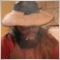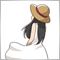# why this shift function dont work?60

``` void Shift(double &array[], double element){

int array_size = ArrayRange(array,0);

for(int j=array_size-1;j>0;j--){array[j] = array[j-1];};

array = element;

```

all indexes positions receive the same value, i think this will be a problem if i make the sort in other direction (array[j+1]=array[j]) but this way i cant find a reason504

```void Shift(double &array[], double element)